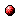# Consistency of fuzzy model-based reinforcement learning

Reference:
L. Busoniu, D. Ernst, B. De Schutter, and R. Babuska, "Consistency of fuzzy model-based reinforcement learning," Proceedings of the 2008 IEEE International Conference on Fuzzy Systems (FUZZ-IEEE 2008), Hong Kong, pp. 518-524, June 2008.

Abstract:
Reinforcement learning (RL) is a widely used paradigm for learning control. Computing exact RL solutions is generally only possible when process states and control actions take values in a small discrete set. In practice, approximate algorithms are necessary. In this paper, we propose an approximate, model-based Q-iteration algorithm that relies on a fuzzy partition of the state space, and on a discretization of the action space. Using assumptions on the continuity of the dynamics and of the reward function, we show that the resulting algorithm is consistent, i.e., that the optimal solution is obtained asymptotically as the approximation accuracy increases. An experimental study indicates that a continuous reward function is also important for a predictable improvement in performance as the approximation accuracy increases.Corresponding technical report: pdf file (537 KB)
Note: More information on the pdf file format mentioned above can be found here.

Bibtex entry:

@inproceedings{BusErn:08-005,
author={L. Bu{\c{s}}oniu and D. Ernst and B. {D}e Schutter and R. Babu{\v{s}}ka},
title={Consistency of fuzzy model-based reinforcement learning},
booktitle={Proceedings of the 2008 IEEE International Conference on Fuzzy Systems (FUZZ-IEEE 2008)},